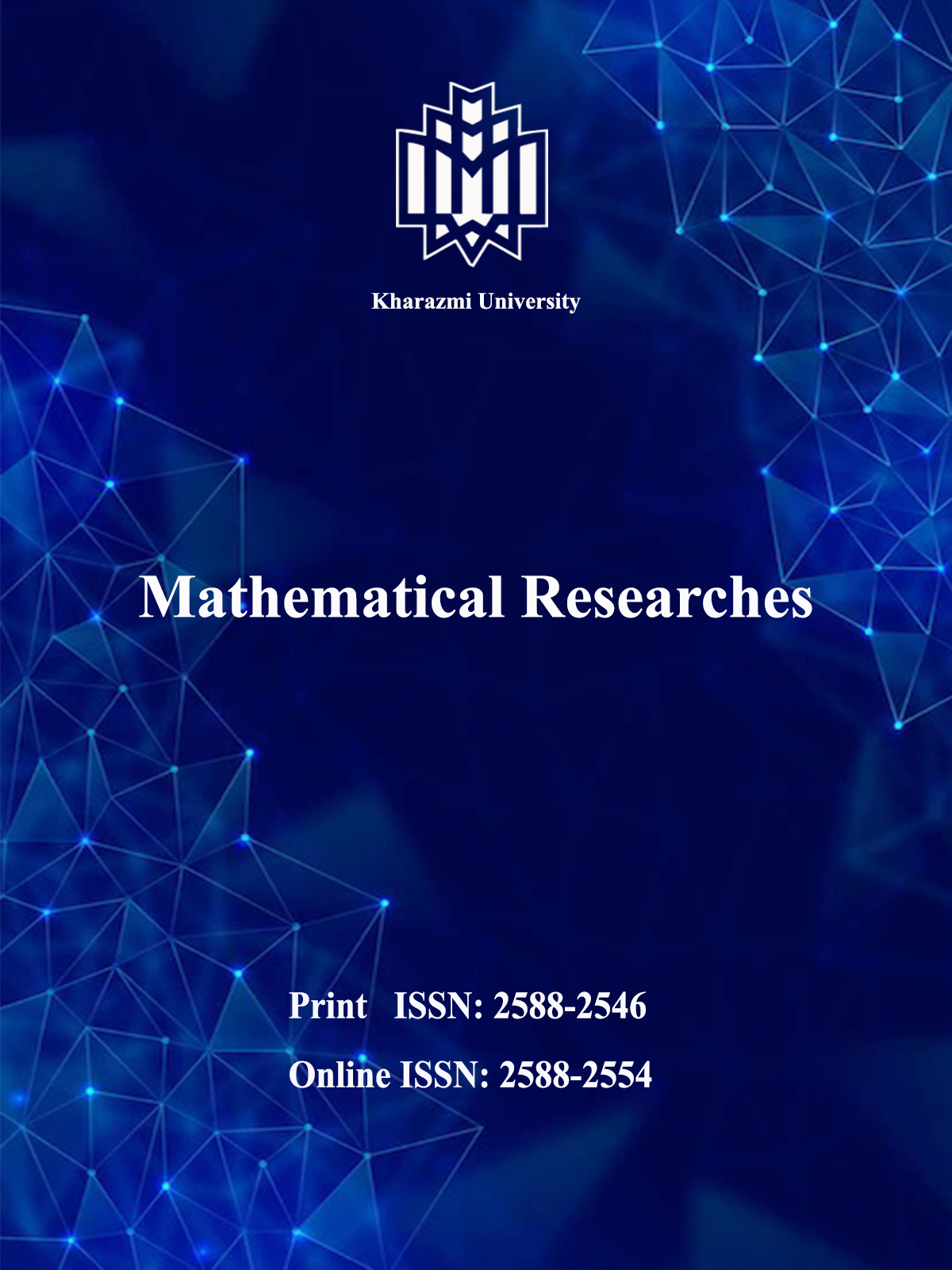[Home ] [Archive]   [ فارسی ]Search Submit ContactHomeJournal InformationArticles archiveFor AuthorsFor ReviewersRegistrationContact usSite FacilitiesEmblem Journal concessionaire  Kharazmi University Managing director Abdol-Javad Taherizadeh, Professor Editor-In-Chief Esmail Babolian, Professor  Manager Marjan Hamdollahi ISSN: 2588-2546 IISSN: 2588-2554Search in website
 Search the articlesSearch the site Advanced SearchEnter your email in the following box to receive the site news and information.Vol. 8, No. 1, 2022#### Mathematical Researches

ISSN Print:    2588-2546 | ISSN Online: 2588-2554
Publisher: Kharazmi UniversityMost visited published articles in last 6 monthsPosynomial geometric programming problem subject to max–product fuzzy relation equationsAn exponential spline for solving the fractional riccati differential equationNumerical solution for the risk of transmission of some novel coronavirus (2019-nCov) models by the Newton-Taylor polynomial solutionsSolving the fractional order integro-differential equations using fractional Jacobi functionsWeak Convergence of Mann Iterative Algorithm for two Nonexpansive mappingsAsymptotic behaviour of graded components of local cohomology modulesInference on Lifetime Performance Index of Two-Parameter Exponential Distribution: Censored and Record DatSome results on fuzzy semi maximal filters in BL-algebrasApplications of quadratic D-forms to generalized quadratic formsSome Properties of the Nil-Graphs of Ideals of Commutative RingsIdentification of outliers types in multivariate time series using genetic algorithmCo-Roman dominating of girdsAn upper bound for the nilpotency class of Leibniz algebrasThe Aluffi Algebra and Linearity ConditionDiagonal Matrix Reduction over Refinement RingsNew methods for constructing shellable simplicial complexesModeling the main and maximum subautomata of an active general fuzzy automatonExistence of multiple solutions for Sturm-Liouville boundary value problemsThe robust vertex centdian location problem with interval vertex weights on general graphsAn improved and efficient stenographic scheme based on matrix embedding using BCH syndrome coding.Wavelet Threshold Estimator of Semiparametric Regression Function with Correlated ErrorsDouble-Frontier Analysis Approach for Weight Derivation in ‎Analytical Hierarchy ProcessA distinct numerical approach for the solution of some kind of initial value problem involving nonlinear q-fractional differential equationsThe (non-)existence of perfect codes in Lucas cubesAn Approach to Deriving Maximal Invariant StatisticsAims| Post date: 2019/08/7 | Aims and Scope The journal of Mathematical Researches (MR) aims to publish high-quality original research articles that make a significant contribution to the research areas of both theoretical and applied mathematics. The journal does not have a length restriction and encourages the submission of longer articles in which more complex and detailed analysis and proofing of theorems are required.  It also publishes shorter research communications (Letters) covering nascent research in some of the hottest areas of mathematical research. The journal of Mathematical Researches (MR) publishes high-quality papers in all of the traditional areas of applied and theoretical areas of mathematics, and it actively seeks to publish seminal papers in the most emerging and interdisciplinary areas in all of the mathematical sciences. The journal of Mathematical Researches (MR) wishes to lead the way by promoting high-quality research of this type. The aim of the journal of Mathematical Researches (MR) is to provide fast publication of refereed, high-quality original research papers in all areas of applied mathematical sciences. This is a broad-based journal covering all branches of mathematics and interdisciplinary research. The journal of Mathematical Researches (MR) publishes research articles that treat scientific problems using methods that are of mathematical interest. Topics of interest include, but are not limited to: Actuarial science.  Algebra and number theory.  Applied mathematics.  Approximation theory and representation theory.  Computational mathematics.  Continuous modeling control theory.   Cryptography.  Differential equations (ODEs and PDEs).  Financial mathematics.  Game theory.   Graph theory.   Information theory.  Linear and nonlinear programming.   Mathematical analysis.  Mathematical biology.   Mathematical economics.   Mathematical methods of engineering.   Mathematical physics.   Mathematics.  Matrix theory.  Numerical analysis.   Operations research.  Optimization.   Probability.   Scientific computing.   Statistics.   Stochastic systems theory.   Theoretical computer science.Vol. 3 No.2 Autumn 2017&Winter 2018.User statistics :. Registered users: 1625 :. Online users: 0 :. Guest users: 10Visits statistics All visits: 1977485 Visits in 24 Hours: 728